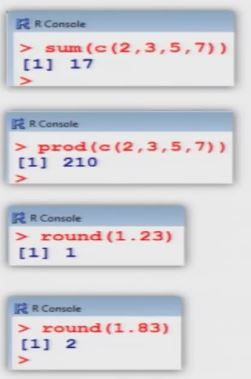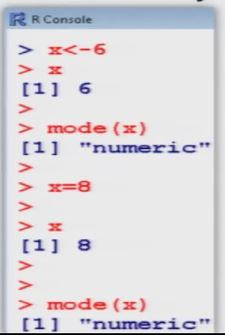# Basics of Calculations_Calculator_Built in Function Assignments

Integer Division %/%

Integer Division :  Division in which the fractional part (remainder) is discarded

> c (2, 3, 5, 7)  % / %  c(2,3)

  1  1  2  2

Modulo Division (x mod y)  %%:

x mod y : modulo operation finds the remainder after division of one number by another

> c (2,3,5,7)  %% 2
  0  1  1  1

Maximum: max

Maximum: min

Overview Over Further Functions

Example :

> abs ( -4)
 4

> abs (c(-1, -2, -3, 4, 5) )
  1  2  3  4  5
> sqrt (4)
  2

> sqrt ( c(4,9,16,25) )
  2  3  4  5> sum (c(2,3,5,7) )
  17

> prod ( c( 2,3,5,7) )
   20

> round  (1.23)
  1

> round (1.83)
  2

Assignments

Assignments can be made in two ways:

>  x<-6>  x
   6

> mode(x)
  "numeric"

> x=8
> x
   8

>  mode (x)
  "numeric"

An assignments can also be used to save values in variables:

>  x1  <-  c(1,2,3,4)

>  x2  <-  x1^2

>  x2
  1  4  9  16

ATTENTION: R is case sensitive (X is not the same as x